# ggplot2: Box Plots

### Introduction

This is the 9th post in the series Elegant Data Visualization with ggplot2. In the previous post, we learnt how to build bar charts. In this post, we will learn to:

• build box plots
• modify box
• color
• fill
• alpha
• line size
• line type
• modify outlier
• color
• shape
• size
• alpha

The box plot is a standardized way of displaying the distribution of data. It is useful for detecting outliers and for comparing distributions and shows the shape, central tendancy and variability of the data.

## Structure

• the body of the boxplot consists of a “box” (hence, the name), which goes from the first quartile (Q1) to the third quartile (Q3)
• within the box, a vertical line is drawn at the Q2, the median of the data set
• two horizontal lines, called whiskers, extend from the front and back of the box
• the front whisker goes from Q1 to the smallest non-outlier in the data set, and the back whisker goes from Q3 to the largest non-outlier
• if the data set includes one or more outliers, they are plotted separately as points on the chart

### Libraries, Code & Data

We will use the following libraries in this post:

All the data sets used in this post can be found here and code can be downloaded from here.

## Data

We are going to use two different data sets in this post. Both the data sets have the same data but are in different formats.

``````daily_returns <- readr::read_csv('https://raw.githubusercontent.com/rsquaredacademy/datasets/master/tickers.csv')
daily_returns``````
``````## # A tibble: 250 x 5
##       AAPL   AMZN      FB    GOOG    MSFT
##      <dbl>  <dbl>   <dbl>   <dbl>   <dbl>
##  1  1.38    24.2   2.12    22.4    1.12
##  2  2.83     3.25 -0.860    5.99   0.767
##  3 -0.0394   9.91  1.45     6.75   0.973
##  4  0.108    3.76 -0.770  -10.7   -0.285
##  5  1.64    19.8   4.75     8.66   0.501
##  6  0.0689   5.33 -0.300   -0.930  0.256
##  7 -0.561   -5.21 -0.630   -7.28  -0.708
##  8  0.551    0.25 -0.460    0.690  0.128
##  9 -0.217  -13.6   0.0300   6.56   0.0786
## 10 -0.108   -4.25  0.460    2.60   0.472
## # ... with 240 more rows``````

## Univariate Box Plot

If you are not comparing the distribution of continuous data, you can create box plot for a single variable. Unlike `plot()`, where we could just use 1 input, in ggplot2, we must specify a value for the X axis and it must be categorical data. Since we are not comparing distributions, we will use `1` as the value for the X axis and wrap it inside `factor()` to treat it as a categorical variable. In the below example, we examine the distribution of stock returns of Apple.

``````ggplot(daily_returns) +
geom_boxplot(aes(x = factor(1), y = AAPL))``````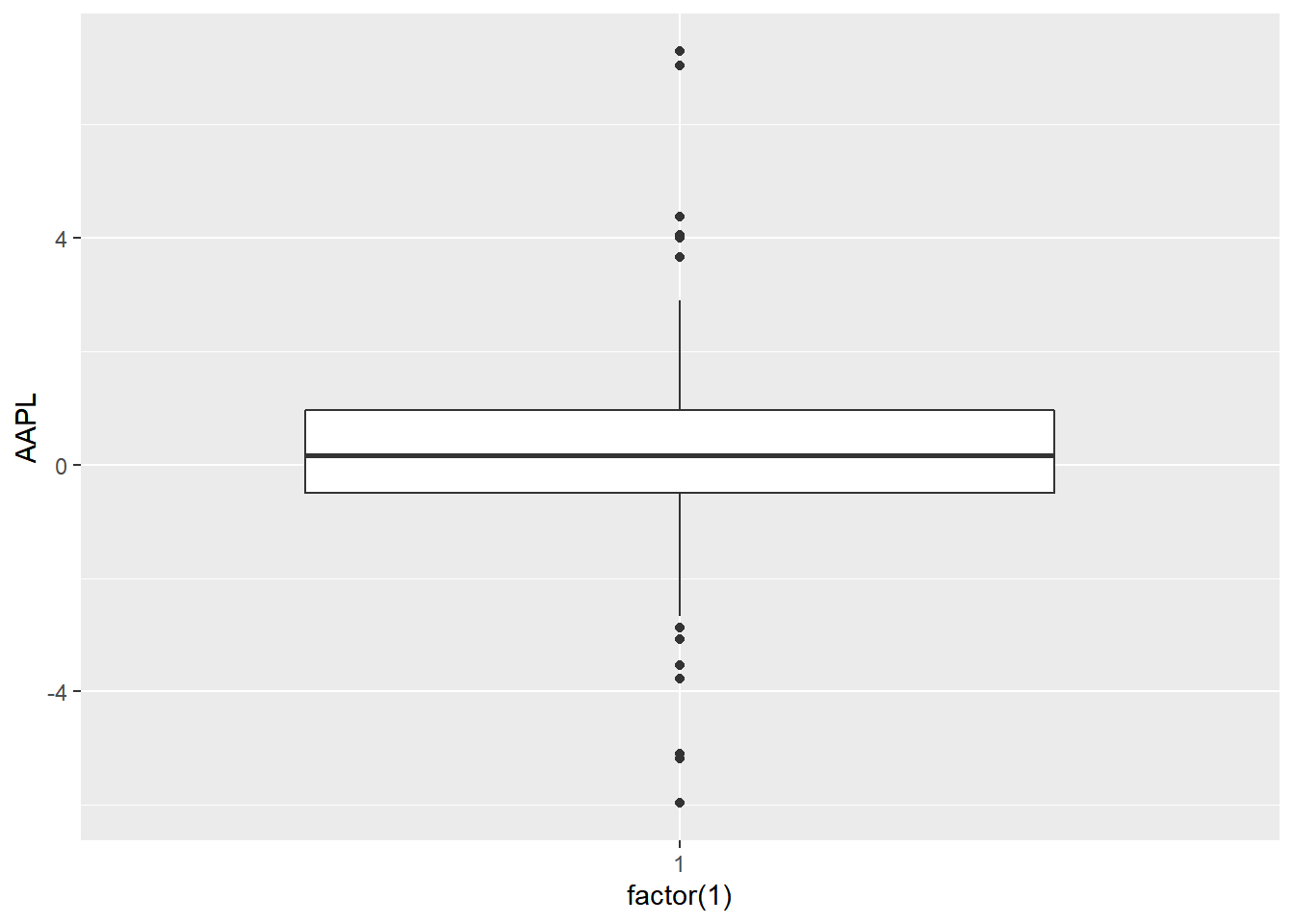## Data

For the rest of the post, we will use the below data set. Instead of 5 columns, we have two columns. One for the stock names and another for returns.

``````tidy_returns <-
col_types = list(col_factor(levels = c('AAPL', 'AMZN', 'FB', 'GOOG', 'MSFT')), col_double()))
tidy_returns``````
``````## # A tibble: 1,254 x 2
##    stock returns
##    <fct>   <dbl>
##  1 AAPL   1.38
##  2 AAPL   2.83
##  3 AAPL  -0.0394
##  4 AAPL   0.108
##  5 AAPL   1.64
##  6 AAPL   0.0689
##  7 AAPL  -0.561
##  8 AAPL   0.551
##  9 AAPL  -0.217
## 10 AAPL  -0.108
## # ... with 1,244 more rows``````

## Box Plot

With the above data, let us create a box plot where we compate the distribution of stock returns of different companies. We map X axis to the column with stock names and Y axis to the column with stock returns. Note that, the column names are wrapped inside `aes()`.

``````ggplot(tidy_returns) +
geom_boxplot(aes(x = stock, y = returns))``````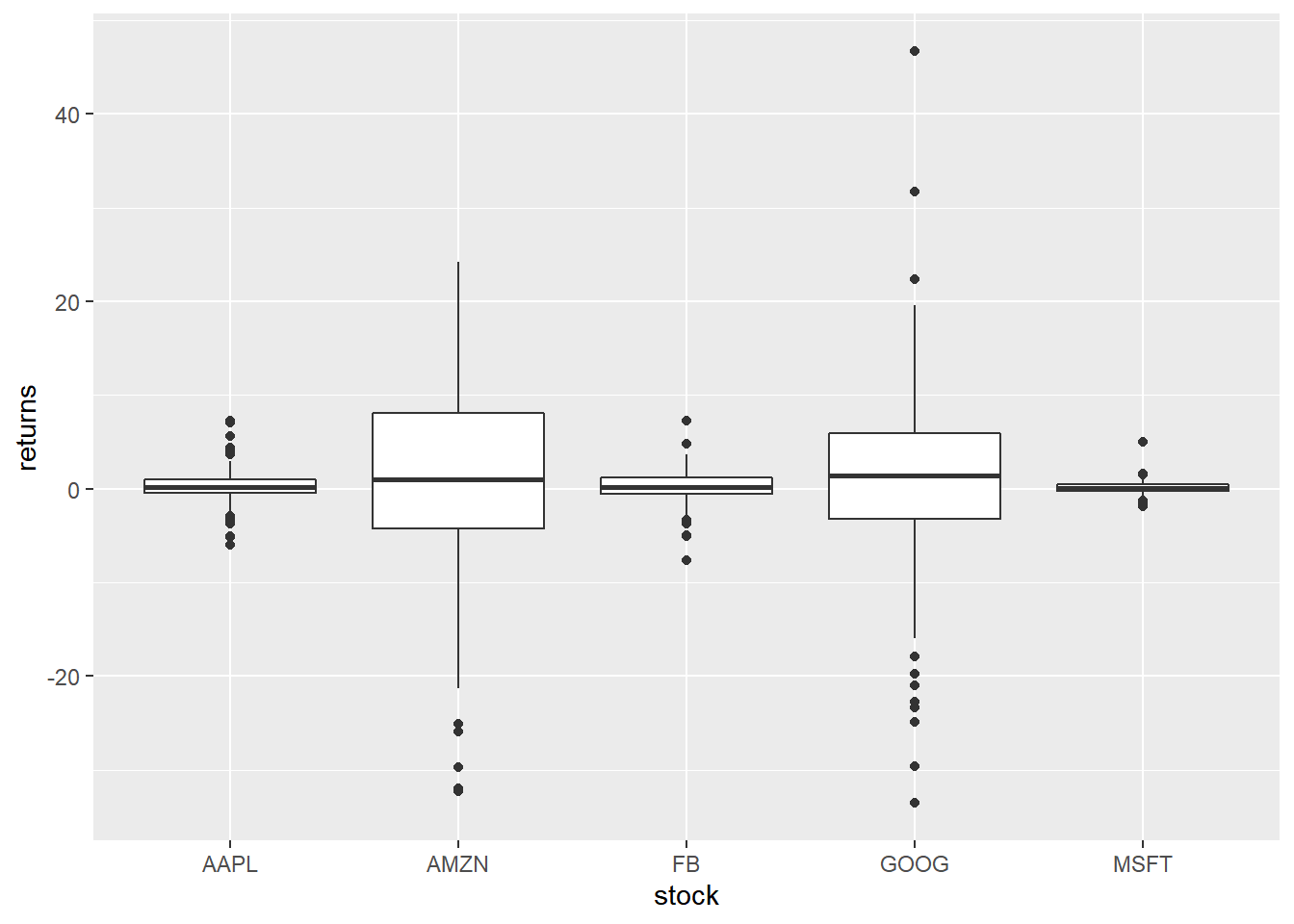To create a horizontal bar plot, we can use `coord_flip()` which will flip the coordinate axes.

## Horizontal Box Plot

``````ggplot(tidy_returns) +
geom_boxplot(aes(x = stock, y = returns)) +
coord_flip()``````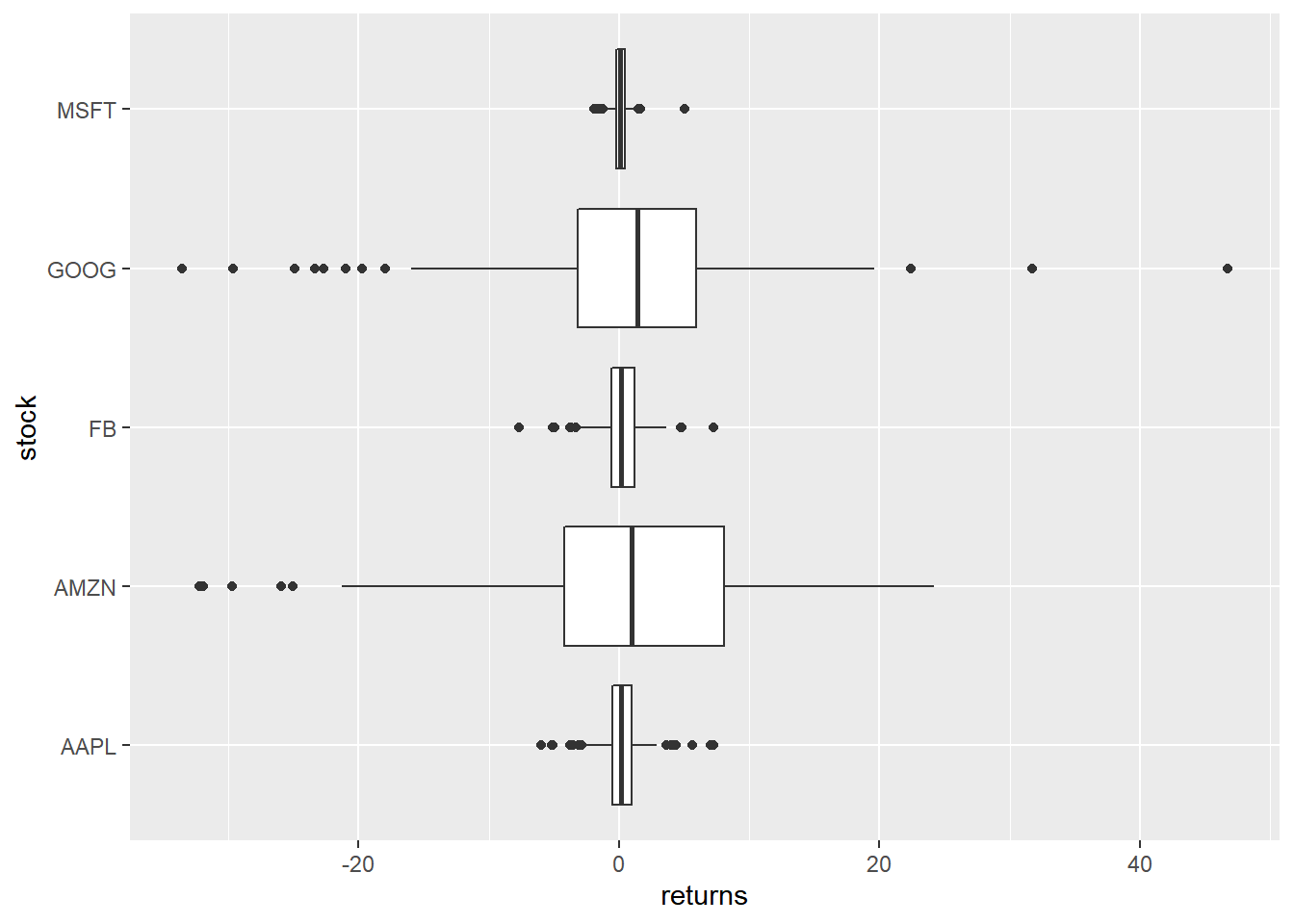## Notch

Notches are used to compare medians. You can use the `notch` argument and set it to `TRUE`.

``````ggplot(tidy_returns) +
geom_boxplot(aes(x = stock, y = returns),
notch = TRUE) ``````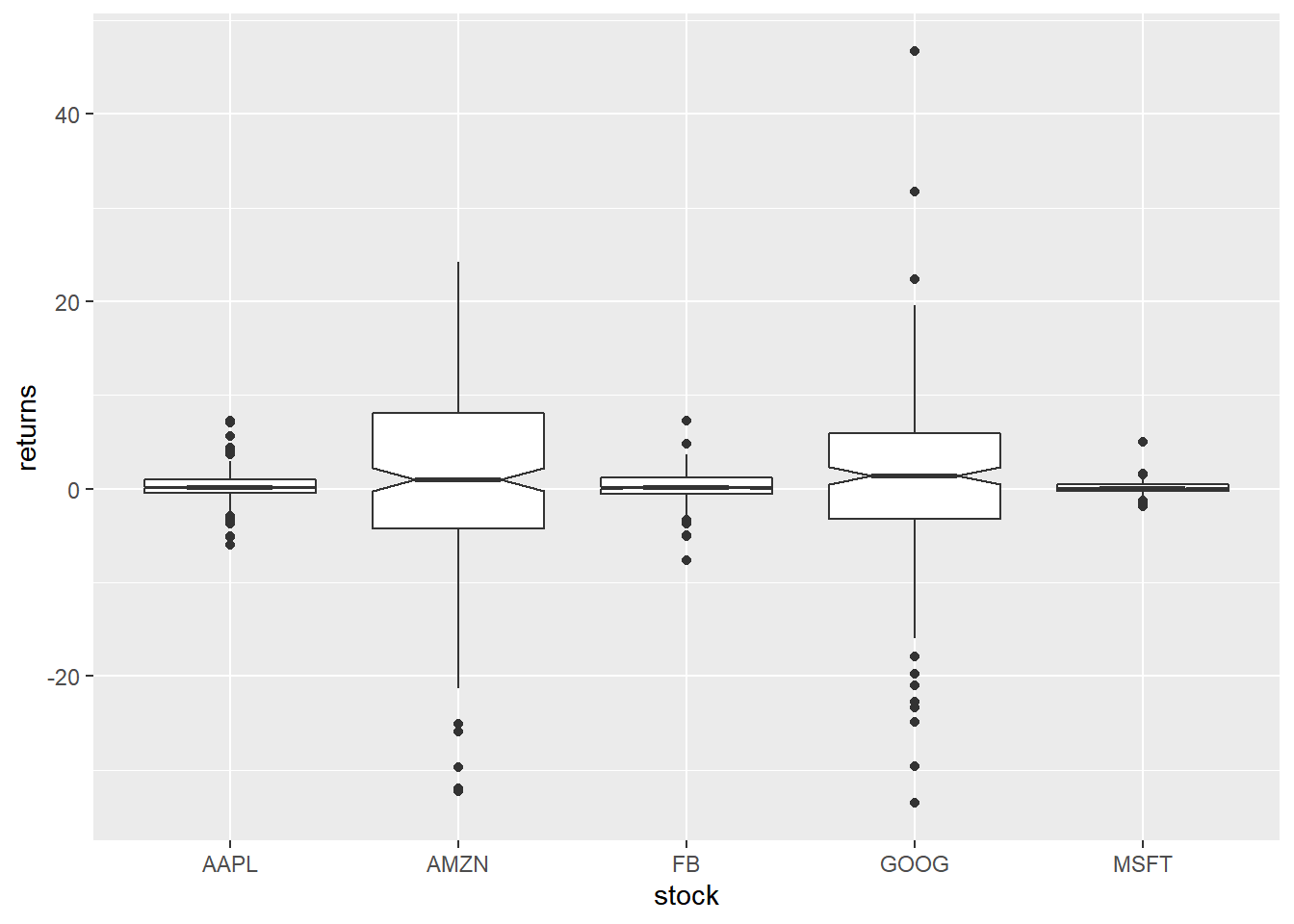## Jitter

Just for comparison, let us plot the returns as points on top of the box plot using `geom_jitter()`. We modify the color of the points using the `color` argument and the spread using the `width` argument.

``````ggplot(tidy_returns, aes(x = stock, y = returns)) +
geom_boxplot() +
geom_jitter(width = 0.2, color = 'blue')``````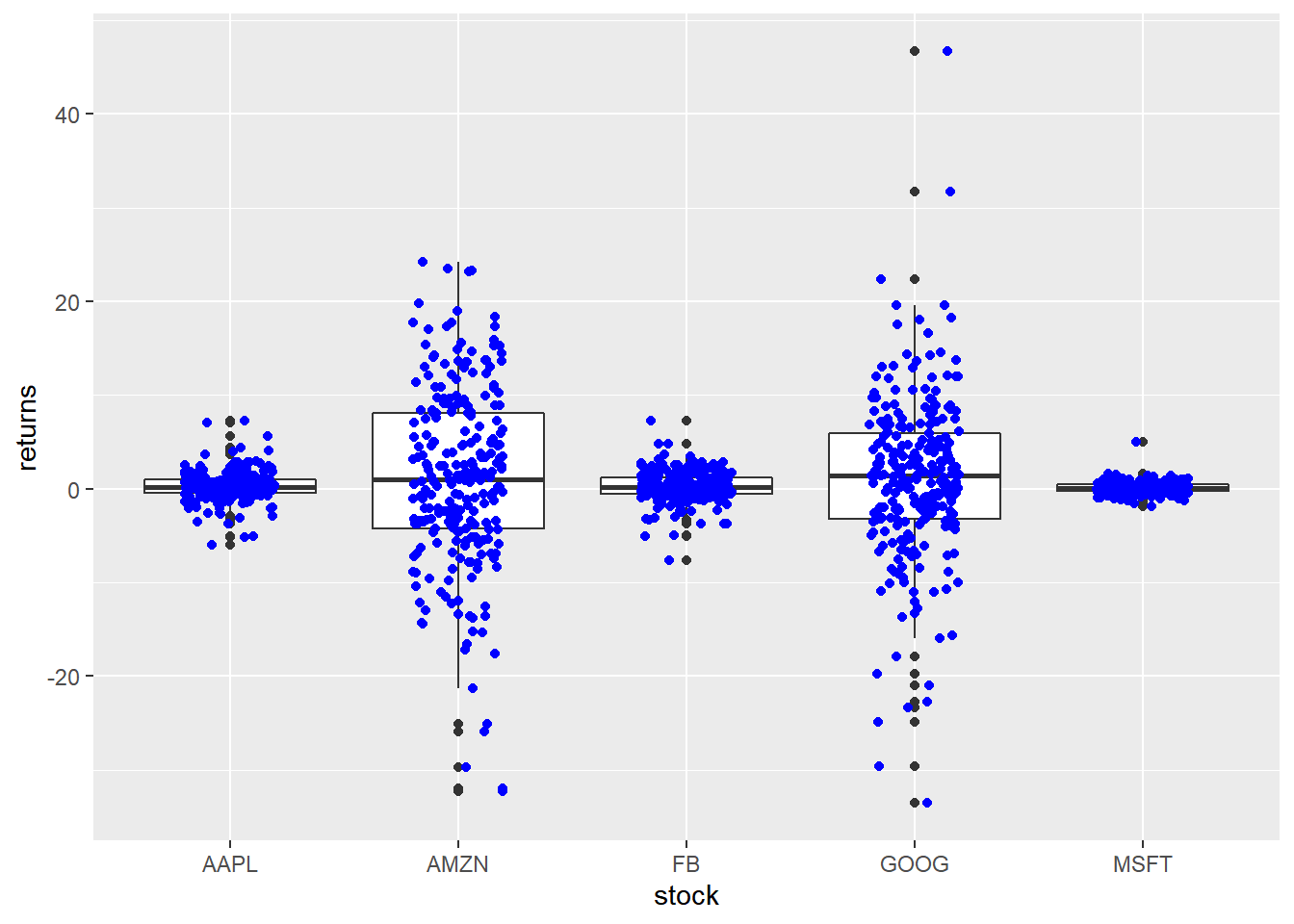## Outliers

To highlight extreme observations, we can modify the appearance of outliers using the following:

• color
• shape
• size
• alpha

To modify the color of the outliers, use the `outlier.color` argument. The color can be specified either using its name or the associated hex code.

``````ggplot(tidy_returns) +
geom_boxplot(aes(x = stock, y = returns), outlier.color = 'red')``````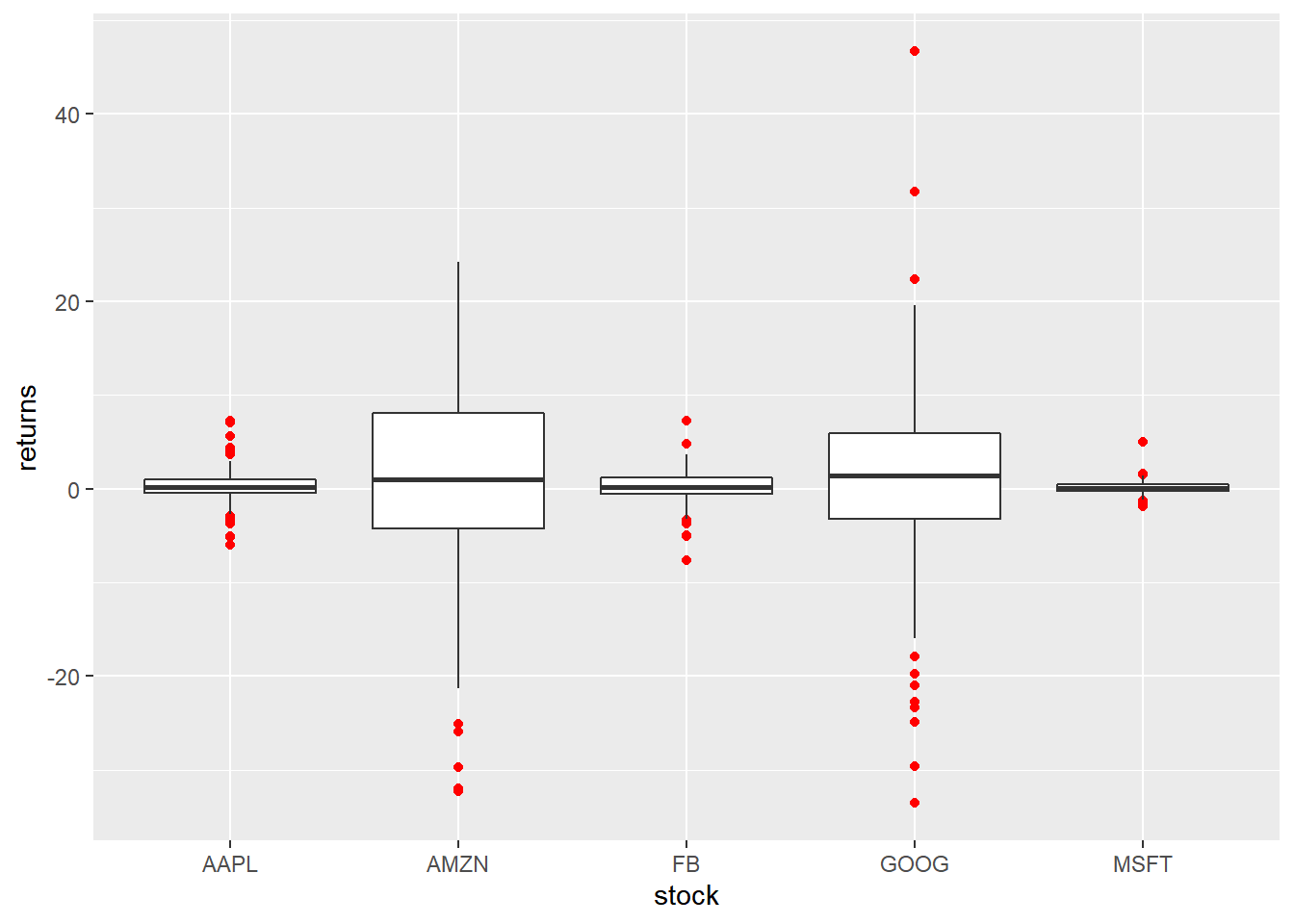The shape of the outlier can be modified using the `outlier.shape` argument. It can take values between `0` and `25`.

``````ggplot(tidy_returns) +
geom_boxplot(aes(x = stock, y = returns), outlier.shape = 23) ``````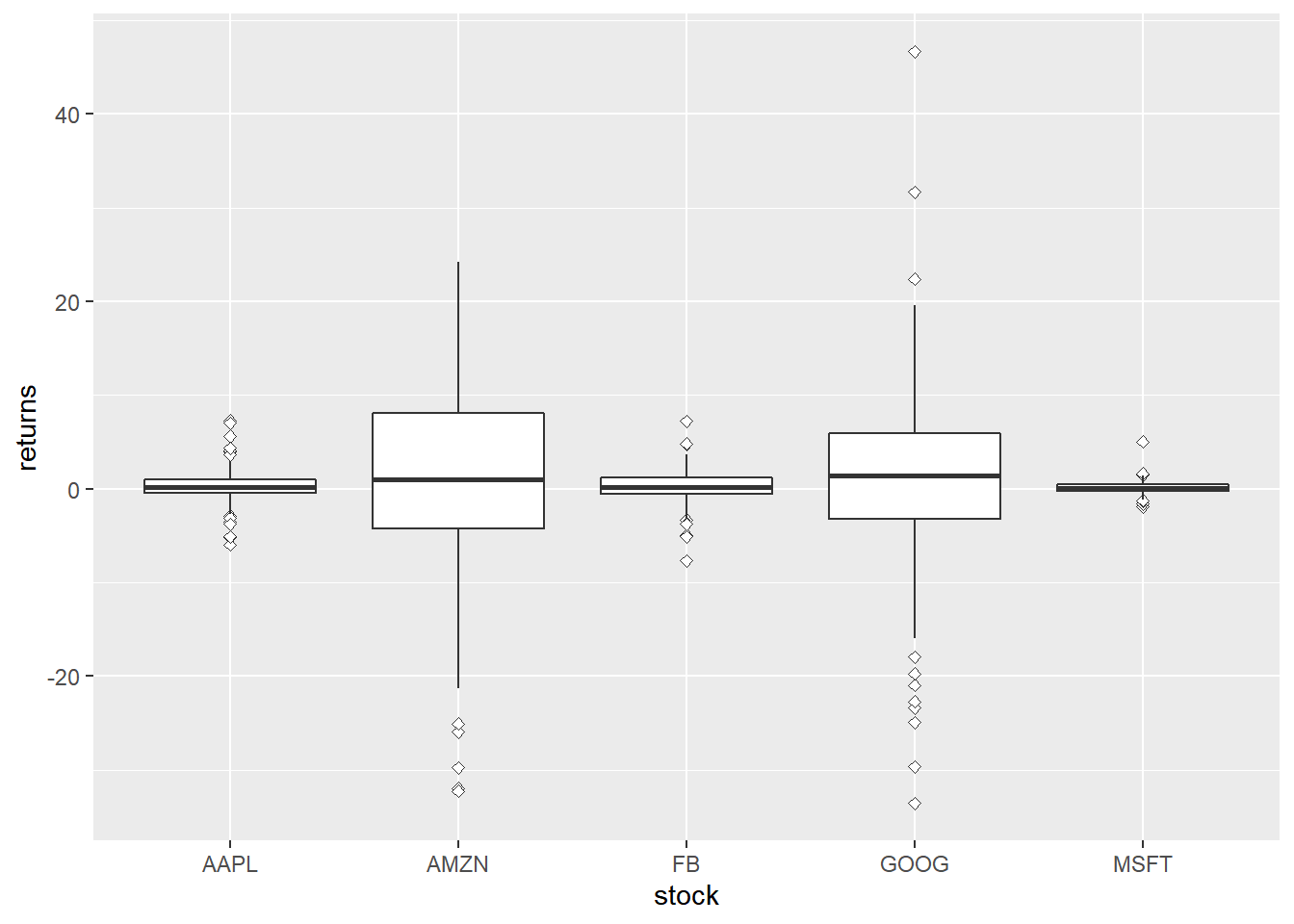The size of the outlier can be modified using the `outlier.size` argument. It can take any value greater than `0`.

``````ggplot(tidy_returns) +
geom_boxplot(aes(x = stock, y = returns), outlier.size = 3) ``````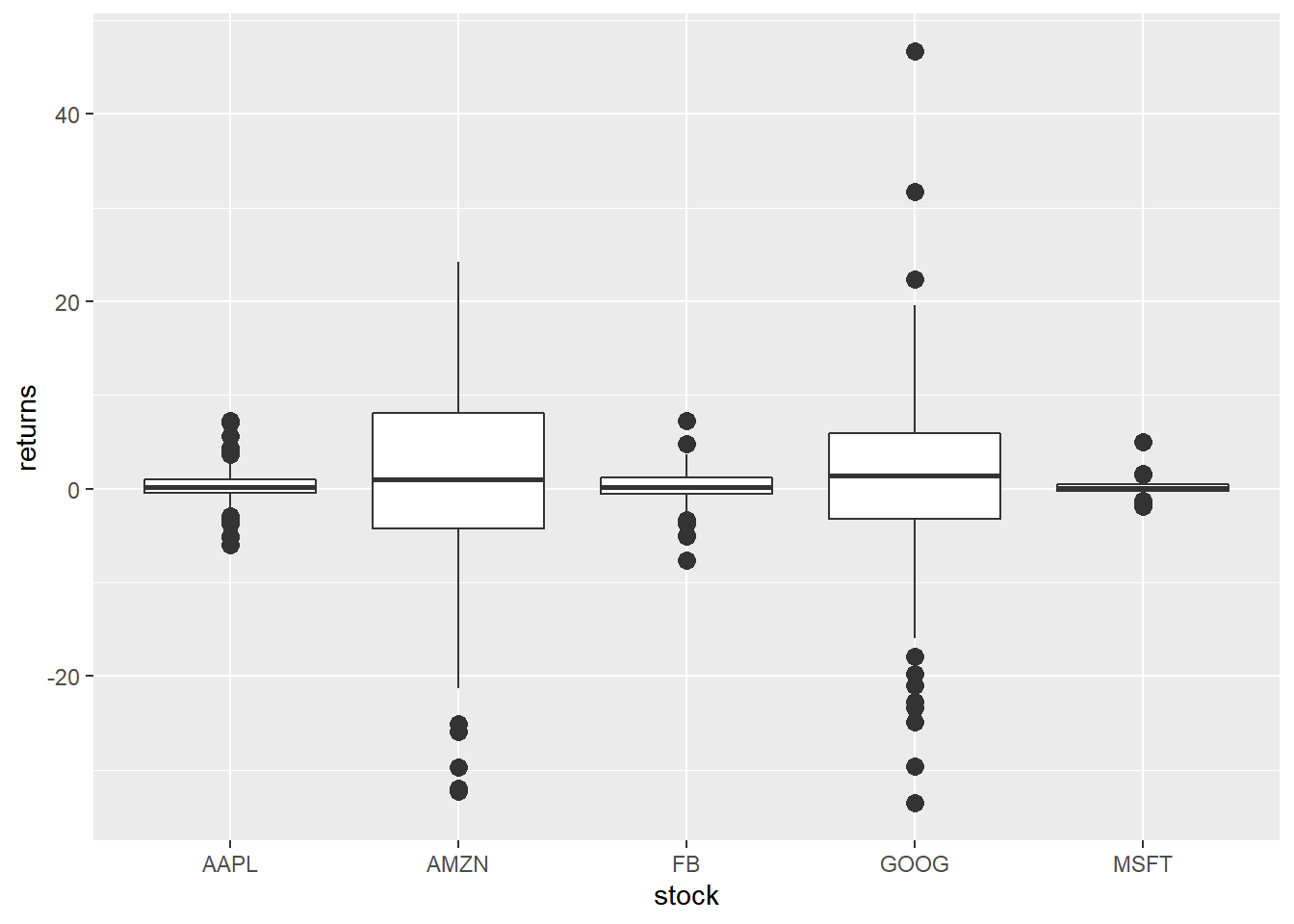You can play around with the transparency of the outlier using the `outlier.alpha` argument. It can take values between `0` and `1`.

``````ggplot(tidy_returns) +
geom_boxplot(aes(x = stock, y = returns), outlier.color = 'blue', outlier.alpha = 0.3) ``````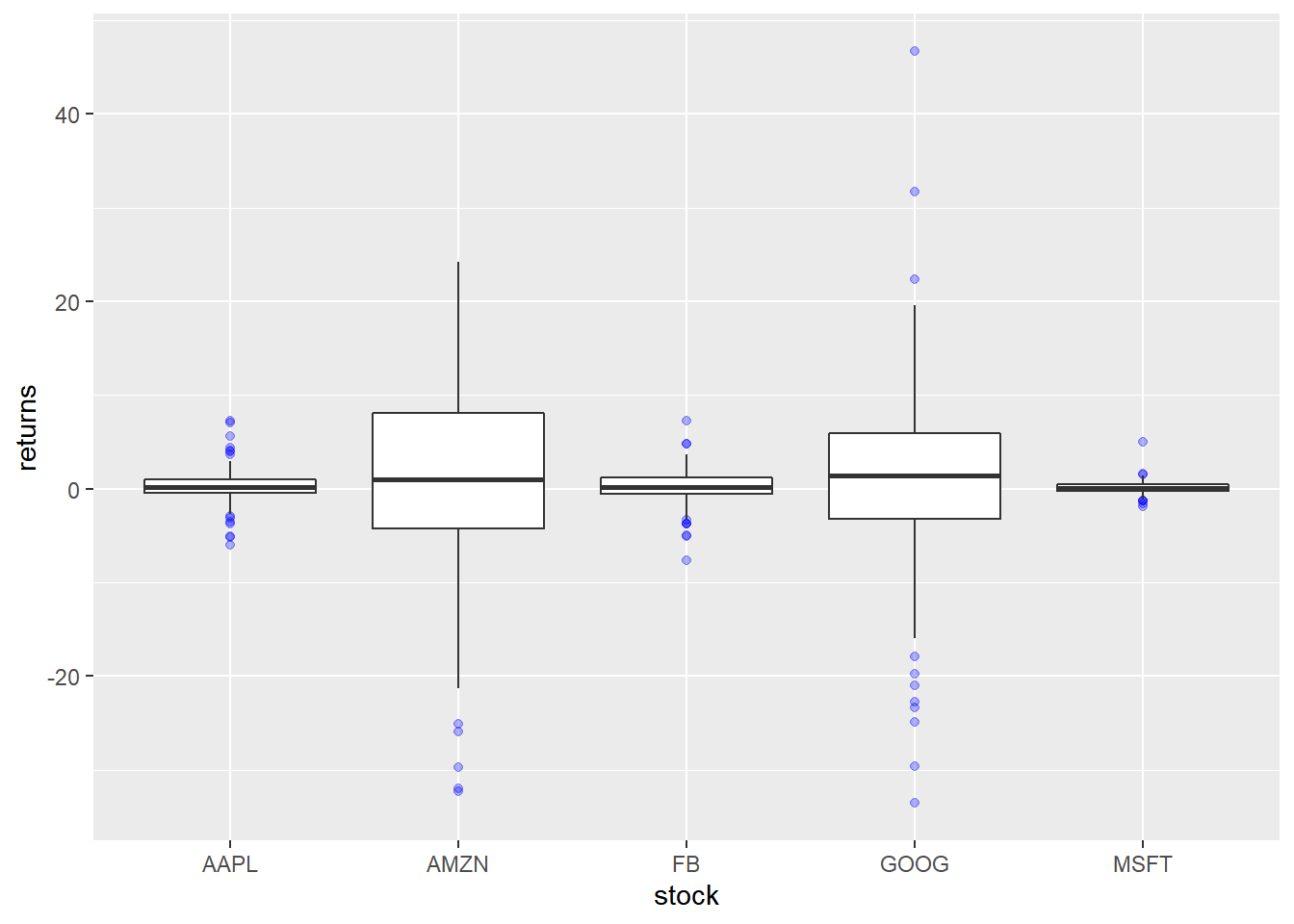## Box Aesthetics

The appearance of the box can be controlled using the following:

• color
• fill
• alpha
• line type
• line width

## Specify Values

The background color of the box can be modified using the `fill` argument. The color can be specified either using its name or the associated hex code.

``````ggplot(tidy_returns) +
geom_boxplot(aes(x = stock, y = returns), fill = c('blue', 'red', 'green', 'yellow', 'brown')) ``````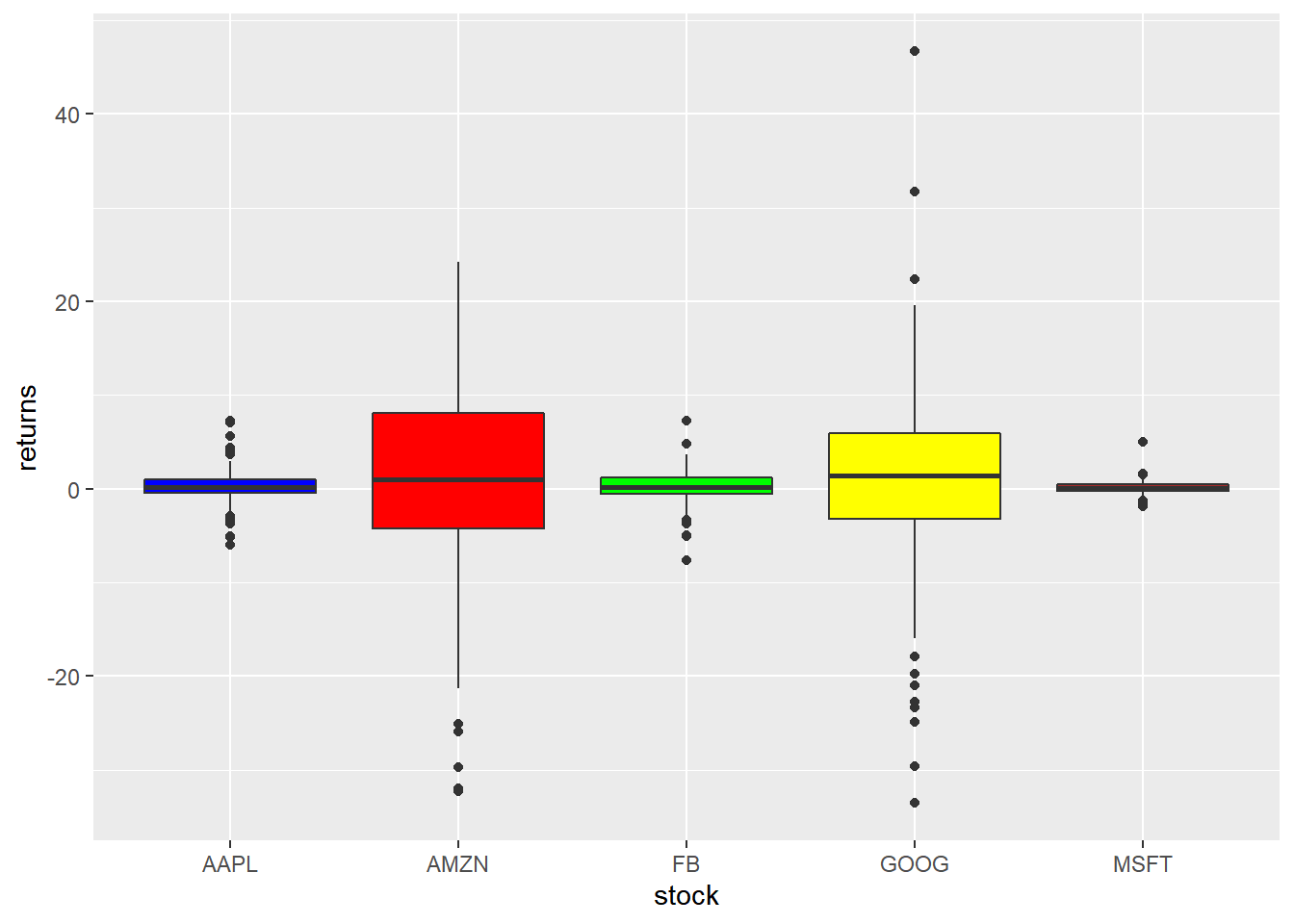To modify the transparency of the background color, use the `alpha` argument. It can take any value between `0` and `1`.

``````ggplot(tidy_returns) +
geom_boxplot(aes(x = stock, y = returns), fill = 'blue', alpha = 0.3) ``````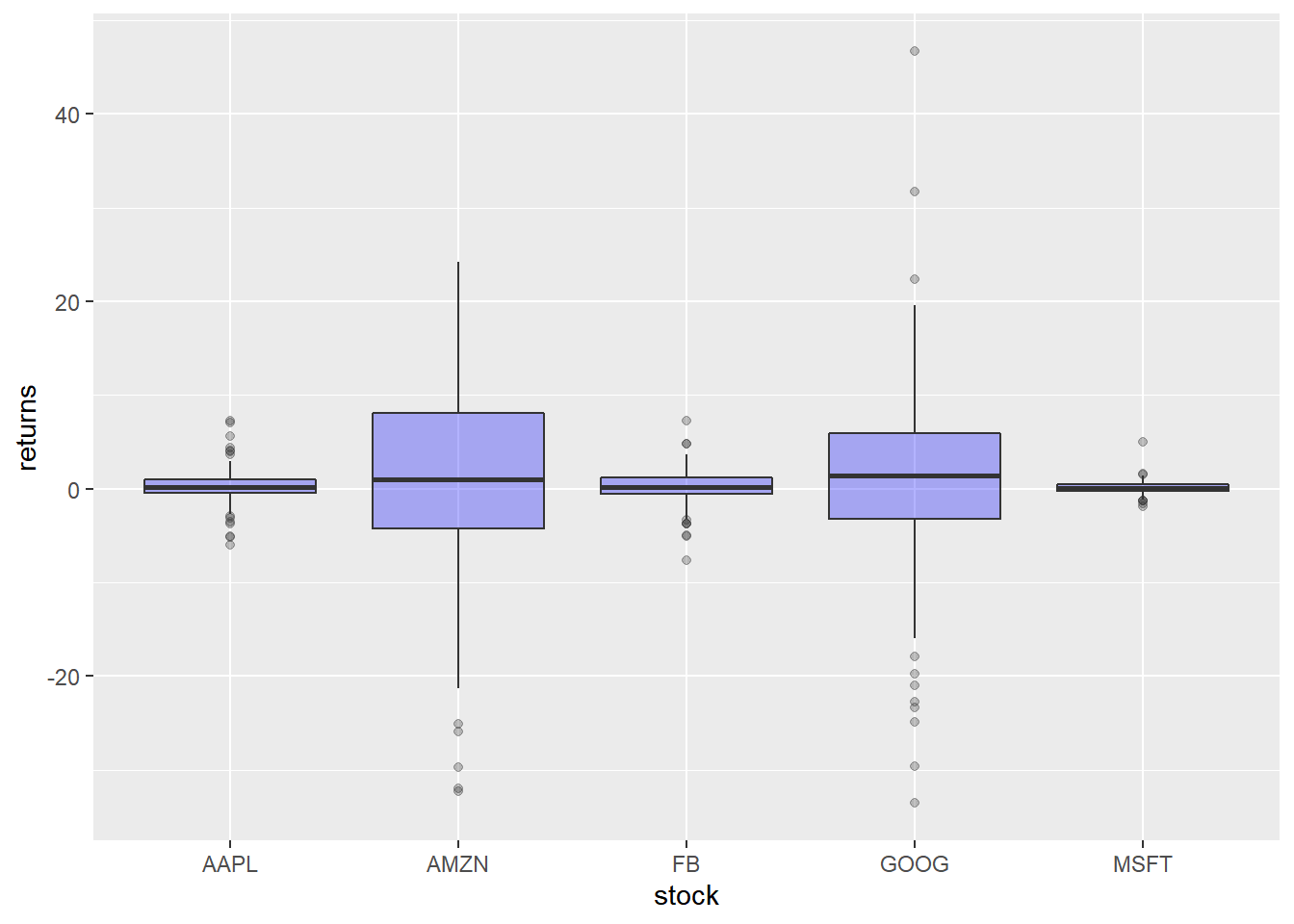The color of the border can be modified using the `color` argument. The color can be specified either using its name or the associated hex code.

``````ggplot(tidy_returns) +
geom_boxplot(aes(x = stock, y = returns), color = c('blue', 'red', 'green', 'yellow', 'brown')) ``````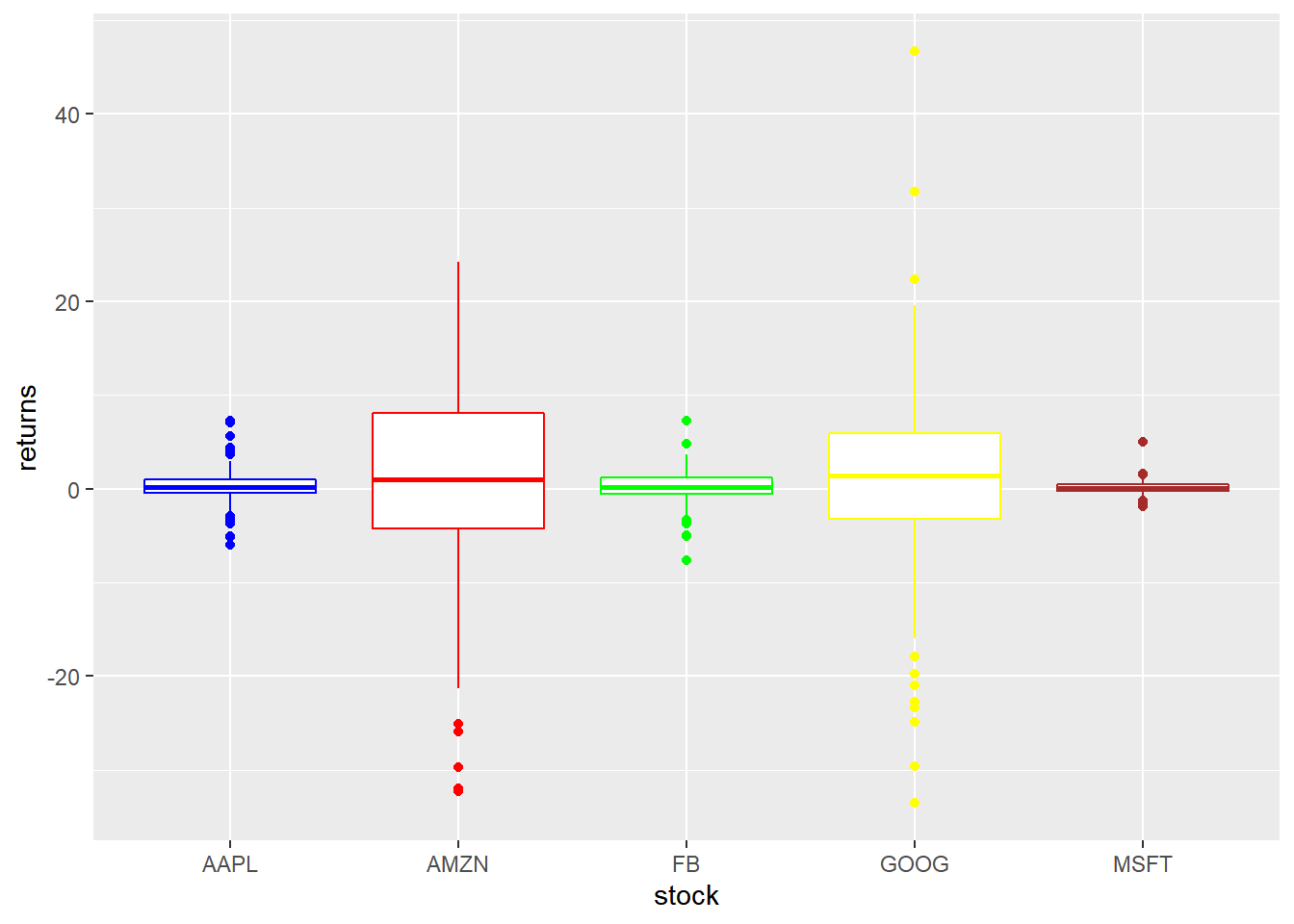The width of the border can be changed using the `size` argument. It can take any value greater than `0`.

``````ggplot(tidy_returns) +
geom_boxplot(aes(x = stock, y = returns), size = 1.5) ``````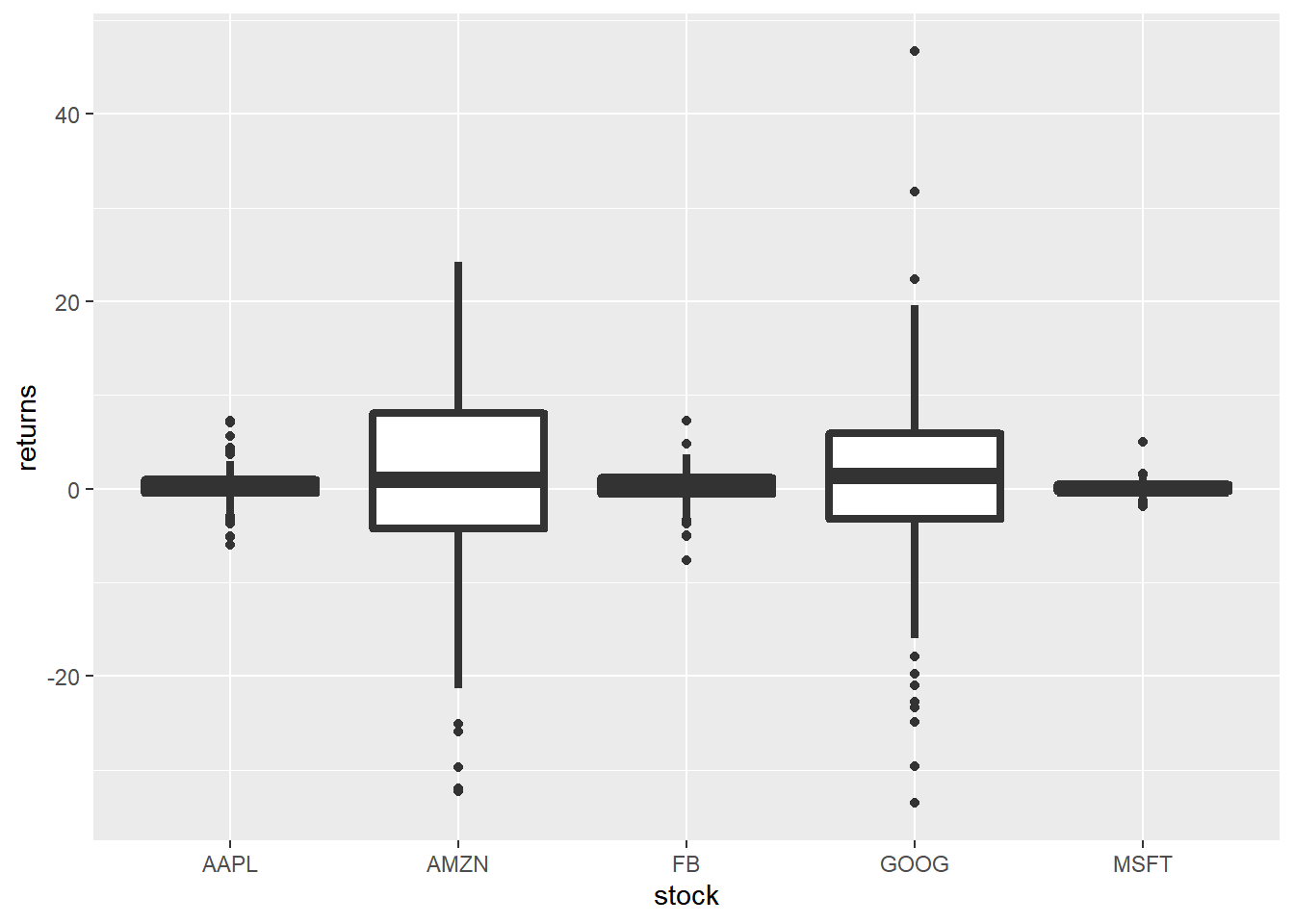To change the line type of the border, use the `linetype` argument. It can take any value between `0` and `6`.

``````ggplot(tidy_returns) +
geom_boxplot(aes(x = stock, y = returns), linetype = 2) ``````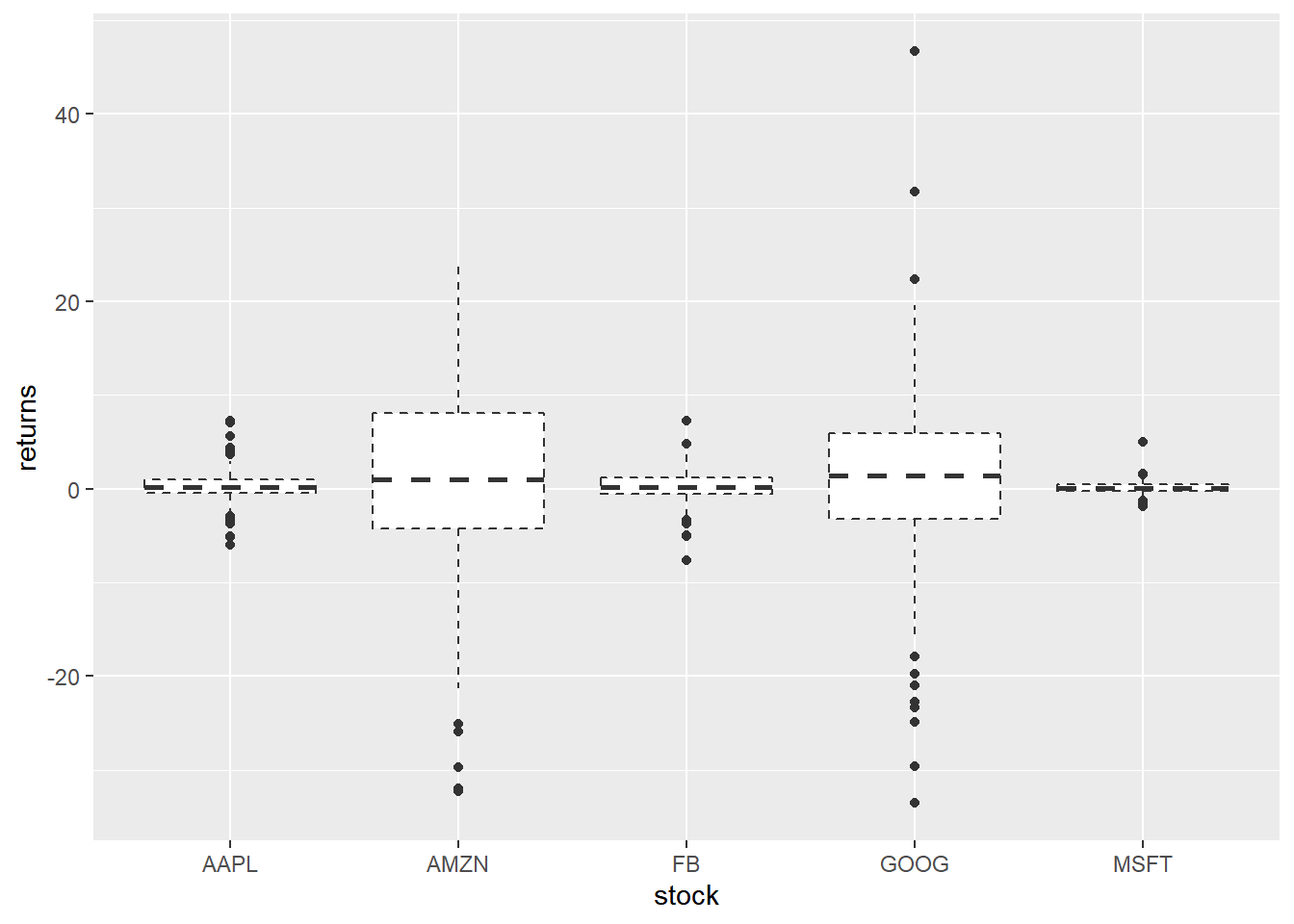## Map Variables

Instead of specifying values, we can map `fill` and `color` to variables as well. In the below example, we map `fill` to the variable stock. It assigns different colors to the different stocks.

``````ggplot(tidy_returns) +
geom_boxplot(aes(x = stock, y = returns, fill = stock)) ``````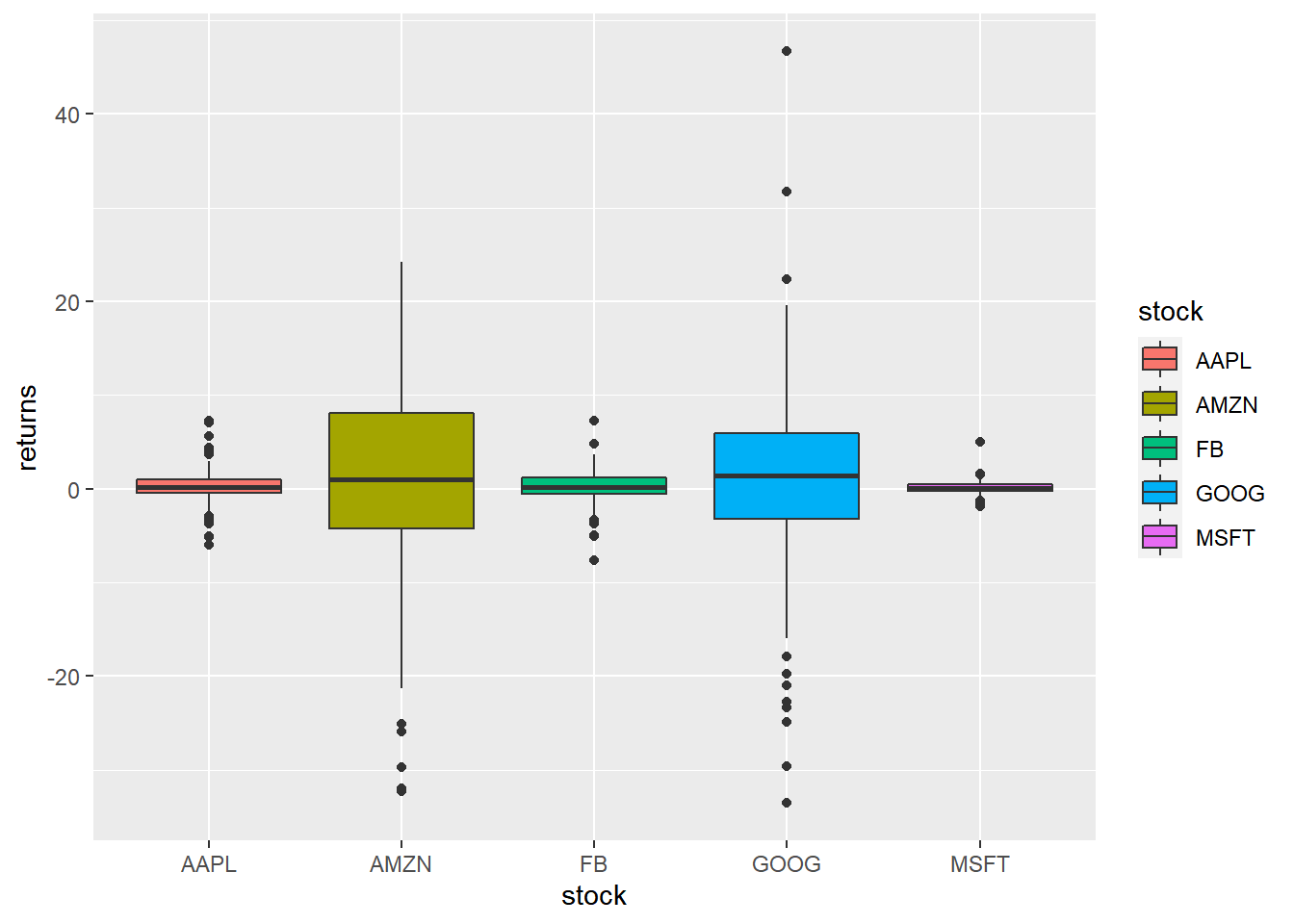Let us map `color` to the variable stock. It will assign different colors to the box borders.

``````ggplot(tidy_returns) +
geom_boxplot(aes(x = stock, y = returns, color = stock)) ``````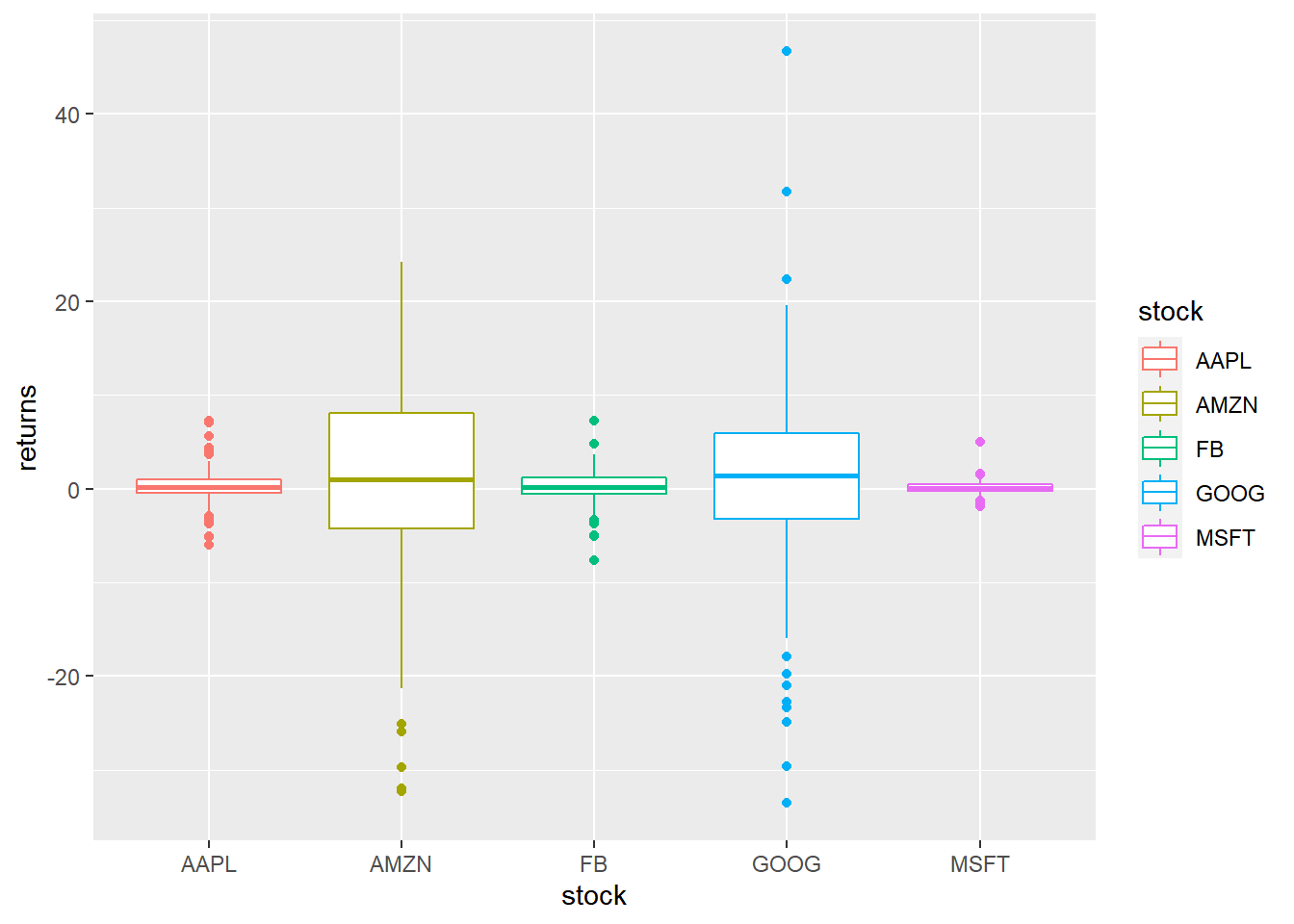### Summary

In this post, we learnt to:

• build box plots
• modify outlier color, shape, size etc.
• modify box color
• modify box line color, size and line type

### Up Next..

In the next post, we will learn to build histograms.